# The parabolic segment

The parabolic segment has a base a = 4 cm and a height v = 6 cm. Calculate the volume of the body that results from the rotation of this segment

a) around its base
b) around its axis.

Correct result:

V1 =  0 cm3
V2 =  50.265 cm3

#### Solution:

$a=4 \ \text{cm} \ \\ v=6 \ \text{cm} \ \\ f(x)=q x^2 \ \\ f(a/2)=q (a/2)^2=v \ \\ 6=q \cdot \ 2^2 \ \\ \ \\ V_{1}=q=6/2^2=\dfrac{ 3 }{ 2 }=1.5=0 \ \text{cm}^3$
$f(x)=v - \dfrac{ 6 }{ 4 } x^2 \ \\ f(x)=6 - \dfrac{ 6 }{ 4 } x^2 \ \\ \ \\ x_{0}=-a/2=-4/2=-2 \ \\ x_{1}=a/2=4/2=2 \ \\ \ \\ V_{2}=\pi \cdot \ \int_\{ x_{0} \}^\{x_{1}\} f(x) dx \ \\ V_{2}=\pi \cdot \ \int_\{x_{0}\}^\{x_{1}\} (\dfrac{ 6 }{ 4 } x^2 - v) dx \ \\ V_{2}=\pi \cdot \ [\dfrac{ 6 }{ 4 } \cdot \ x^3/3 - vx]_\{x_{0}\}^\{x_{1}\} \ \\ \ \\ F(x)=6x - \dfrac{ 6 }{ 4 } \cdot \ x^3/3 \ \\ F_{1}=6 \cdot \ x_{1} - \dfrac{ 6 }{ 4 } \cdot \ x_{1}^3/3=6 \cdot \ 2 - \dfrac{ 6 }{ 4 } \cdot \ 2^3/3=8 \ \\ F_{0}=6 \cdot \ x_{0} - \dfrac{ 6 }{ 4 } \cdot \ x_{0}^3/3=6 \cdot \ (-2) - \dfrac{ 6 }{ 4 } \cdot \ (-2)^3/3=-8 \ \\ \ \\ V_{2}=\pi \cdot \ (F_{1} - F_{0})=3.1416 \cdot \ (8 - (-8))=50.265 \ \text{cm}^3$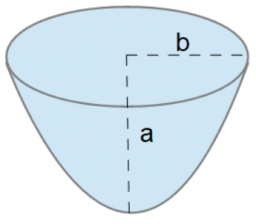Our examples were largely sent or created by pupils and students themselves. Therefore, we would be pleased if you could send us any errors you found, spelling mistakes, or rephasing the example. Thank you!

Leave us a comment of this math problem and its solution (i.e. if it is still somewhat unclear...):Be the first to comment!#### You need to know the following knowledge to solve this word math problem:

We encourage you to watch this tutorial video on this math problem:

## Next similar math problems:

• The tentCalculate how much cover (without a floor) is used to make a tent that has the shape of a regular square pyramid. The edge of the base is 3 m long and the height of the tent is 2 m.
• Triangular prismCalculate the surface of a regular triangular prism, the edges of the base are 6 cm long and the height of the prism is 15 cm.
• Pentagonal prismThe regular pentagonal prism is 10 cm high. The radius of the circle of the described base is 8 cm. Calculate the volume and surface area of the prism.
• The basesThe bases of the isosceles trapezoid ABCD have lengths of 10 cm and 6 cm. Its arms form an angle α = 50˚ with a longer base. Calculate the circumference and content of the ABCD trapezoid.
• Two hemispheres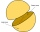In a wooden hemisphere with a radius r = 1, a hemispherical depression with a radius r/2 was created so that the bases of both hemispheres lie in the same plane. What is the surface of the created body (including the surface of the depression)?
• Garden exchangeThe garden has the shape of a rectangular trapezoid, the bases of which have dimensions of 60 m and 30 m and a vertical arm of 40 m. The owner exchanged this garden for a parallelogram, the area of which is 7/9 of the area of a trapezoidal garden. What is
• Annulus from triangleCalculate the content of the area bounded by a circle circumscribed and a circle inscribed by a triangle with sides a = 25mm, b = 29mm, c = 36mm
• Wooden box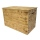The block-shaped box was placed on the ground, leaving a rectangular print with dimensions of 3 m and 2 m. When flipped over to another wall, a print with dimensions of 0.5 m and 3 m remained in the sand. What is the volume of the wooden box?
• Alcohol from potatoes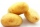In the distillery, 10 hl of alcohol can make from 8 t of potatoes. The rectangular field with dimensions of 600 m and 200 m had a yield of 20 t of potatoes per hectare. How many square meters of area are potatoes grown to obtain one liter of alcohol?
• Two sides paintThe door has the shape of a rectangle with dimensions of 260cm and 170cm. How many cans of paint will be needed to paint this door if one can of paint cover 2m2 of the area? We paint the doors on both sides.
• Largest squaresHow many of the largest square sheets did the plumber cut the honeycomb from 16 dm and 96 dm?
• FlakesA circle was described on the square, and a semicircle above each side of the square was described. This created 4 "flakes". Which is bigger: the content of the central square or the content of four chips?
• A mapA map with a scale of 1: 5,000 shows a rectangular field with an area of 18 ha. The length of the field is three times its width. The area of the field on the map is 72 cm square. What is the actual length and width of the field?
• Rectangular land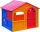On a rectangular land with dimensions of 35 m and 18.5 m is a house with a square floor plan with a side of 14 m. What % of the land is not occupied?
• Compute 4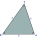Compute the exact value of the area of the triangle with sides 14 mi, 12 mi, and 12 mi long.
• Two gardens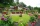The total area of the two gardens is 864 m2. The first garden is 60 m2 smaller than three times the second garden. What is the area of each garden?
• Playground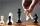On the special playground, there are 81 square sectors, each with a side of 5 m. How many players can fit on the playground if each player needs a 75 m2 area to play?